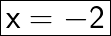## What is the value of x in the solution to the system of equations? 4x – 2y = 5 y = 3x – 1​

Question

What is the value of x in the solution to the system of equations? 4x – 2y = 5 y = 3x – 1​

in progress 0
6 months 2021-09-05T00:36:32+00:00 2 Answers 6 views 0Step-by-step explanation:

4x – 2y = 5 ——————————-(1)

y = 3x – 1 ———————————-(2)

Put Eq. (2) in (1)

4x – 2(3x-1) = 5

4x – 6x + 1 = 5

-2x + 1 = 5

Subtracting 1 to both sides

-2x = 5 – 1

-2x = 4

Dividing both sides by -2

x = 4 / -2

x = -2Hope this helped!